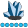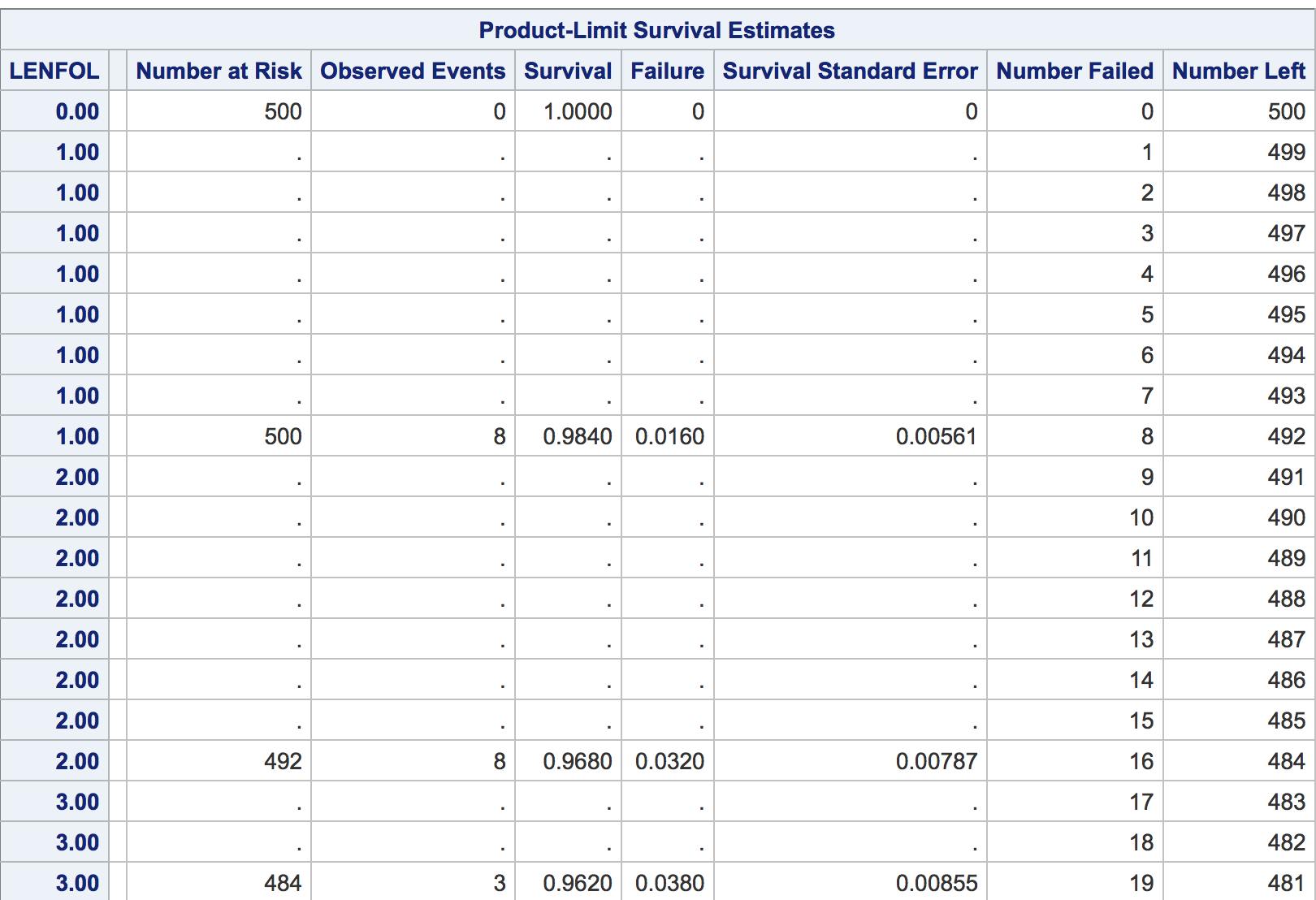## PROC PHREG - survival probability at specific point of time

Hello,

I'm making a model in SAS 4GL using PROC PHREG procedure. In the output out statement it is possible to define a survival variable for each observation. This survival variable is the probability of survival until some point of time. My question is: what time is considered here? Is it a probability of surviving until time=0?

Is it possible to get a variable like this but describing a probability of surviving until some arbitraty defined point of time? (i.e. 1 year?)

My code is following:

```ods graphics on;
proc phreg data=train outest=estimates plots=(survival cumhaz);
class &vars;
model time*censor(1)=&vars / selection=stepwise ties=efron;
output out=surv survival=s;
run;
ods graphics off;```

8 REPLIES 8DarthPathos
Lapis Lazuli | Level 10

## Re: PROC PHREG - survival probability at specific point of time

Hi @klaur - hope you're having a good weekend!

I have done a lot of survival analysis but I admit i've only ever used PROC LIFETEST.  One of the reasons is that it can generate a table such as the one i think you're looking for; screenshot below taken from here.  You can output this table as a SAS dataset so you can use it for other purposes.  Looking down the "Survival" column, I can find the point when 80% are still alive (or 20% failed).

Hopefully this will help; please post back and let me know if you need anything further.

ChrisHas my article or post helped? Please mark as Solution or Like the article!

## Re: PROC PHREG - survival probability at specific point of time

Thank you for the reply! Unfortunately it's not really what I'm looking for. I'm making a Cox proportional hazards model so I need to use proc phreg procedure.

Plus what I want to obtain is a variable for each of the observations that tells me 'the probability of survival for this observation until let's say 1 year is something'.

## Re: PROC PHREG - survival probability at specific point of time

Since you have a model that's more complex than just a basic KM curve, you may also need to consider the values of the covariates when you're trying to calculate survival. If you're including the variables you have some belief that these affect survival so the estimates would be different give the values of the covariates.

The method to calculate the survival estimate is to use the BASELINE statement and specify the level of the covariates. The default is to use the Mean for continuous variables and the reference level for categorical variables.

See the documentation example labeled 'Survival Curves' in combination with the data behind the graphs if you want it as well.

http://support.sas.com/documentation/cdl/en/statug/68162/HTML/default/viewer.htm#statug_phreg_exampl...

http://blogs.sas.com/content/iml/2012/08/01/data-fro-ods-graphics.html

## Re: PROC PHREG - survival probability at specific point of time

I know there is a possibility to use the baseline statement where I can define the specific covariates. However my purpose is not to see the survival just for some specific combination of covariates. I would like to see the estimated survival for each of my observations for the specific point of time.

Do you perhaps know what is the interpretation of the survival 's' I get in the output dataset when I use 'output out=dataset survival=s'. It is a probability of surviving but until what time?

## Re: PROC PHREG - survival probability at specific point of time

Hello,

I think I have the same issue as Klaur does. I'm working to get 5-year survival probability for each patinet in the dataset, and for that first I identified the So(t) by using the Baseline statement and setting the values of all covriates at ZERO, and then I looked into the dataset and took the So(5_y) which was the survival function corresponding to Time equals 5 years (or near to 5 years if I didn't have any value exactly equaled 5 years). Using the formual S(t)=So(t)^exp(XB) I could get the 5-year survival probab for each individual.

BUT I want to compare my findings with those generated by a program in SAS, and see if SAS gives me the same 5-year survival probablity for each individual in my dataset. So far I couldn't figure it out, as there is no way I can specify time=5 in SAS, and it gives error. I appreciate if someone can help on this.

proc phreg data=Mylib.dataset;

class group (ref ='1') category (ref ='1')/ param=ref;

model time (time=5)*event(0)= group category age;

baseline covariates=Mylib.dataset OUT=Pred1 survival=_all_;

run;

## Re: PROC PHREG - survival probability at specific point of time

Have you checked the dataset created by OUT option on BASELINE statement or the TIMELIST option?

## Re: PROC PHREG - survival probability at specific point of time

Hi, were you able to find out how to produce estimated probabilities from proc phreg?

Thanks

FlorenciaDarthPathos
Lapis Lazuli | Level 10

## Re: PROC PHREG - survival probability at specific point of time

HI Vivapoe! Apologies for the delay, have you seen the link https://support.sas.com/documentation/cdl/en/statug/63033/HTML/default/viewer.htm#statug_phreg_sect0... - it might get you started trying to figure estimated probabilities.  Post back if you have any other questions (and I'll try to be quicker in replying! :-))

have a great day!

Chris

Has my article or post helped? Please mark as Solution or Like the article!
Discussion stats
• 8 replies
• 8532 views
• 1 like
• 5 in conversation